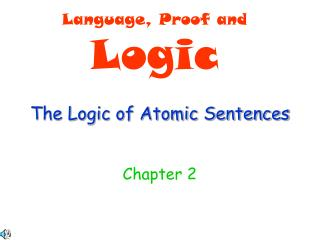Download PresentationThe Logic of Atomic SentencesThe Logic of Atomic Sentences - PowerPoint PPT Presentation

Download PresentationThe Logic of Atomic Sentences
An Image/Link below is provided (as is) to download presentation

Download Policy: Content on the Website is provided to you AS IS for your information and personal use and may not be sold / licensed / shared on other websites without getting consent from its author. While downloading, if for some reason you are not able to download a presentation, the publisher may have deleted the file from their server.

- - - - - - - - - - - - - - - - - - - - - - - - - - - E N D - - - - - - - - - - - - - - - - - - - - - - - - - - -
Presentation Transcript

1. Language, Proof and Logic The Logic of Atomic Sentences Chapter 2

2. 2.1a Valid and sound arguments • Anargumentis a series of statements in which one, called the • conclusion, is meant to be a consequence of the others, called the • premises. • An argument is valid if the conclusion must be true in any circumstance • in which the premises are true. We say that the conclusion of a valid • argument is a logical consequence of its premises. • An argument is soundif it is valid and the premises are true. • Is the following argument valid? Is it also sound? Premises: All humans have two legs Teddy is a human Conclusion: Teddy has two legs

3. 2.1b Valid and sound arguments The Fitch format: All humans have two legs Teddy is a human Teddy has two legs

4. 2.2a • To show that an argument is invalid, you need to find a counterexample, • i.e. a circumstance that makes all premises true but the conclusion false. • To show that an argument is valid, you need to find a proof, i.e. a • step-by-step demonstration that the conclusion follows from the premises. • Usually involves many intermediate steps. • Informal proof: Usually uses English perhaps intermixed with symbolic • expressions. Lazily combines series of “obvious” steps. All proofs that • you have seen in geometry (or elsewhere) are informal. • Formal proof: Follows a strictly predefined set of rules, such as, say, • 1. Cube(c) • 2. c=b • 3. Cube(b)= Elim: 1,2 Methods of proof

5. 2.2b Rigor: At every step in the proof, the conclusion should follow from the premises with absolute certainty. All formal proofs are, of course, rigorous. But so can and should be (good) informal proofs. Why rigor is necessary: Imagine the steps/transitions relied upon only provide 99% certainty. What would be the probability that the conclusion indeed follows from the premises if the number of steps in the proof is: 1? 2? 10? 100? 1000000? Methods of proof 99% about 98% about 90% about 35% practically 0%

6. 2.2c • = Elim (indiscernibility of identicals): • If b=c, then whatever holds of b also holds of c. • = Intro (reflexivity of identity): • Sentences of the form b=b are always true. • 3. Symmetry of identity: If b=c, then c=b. • 4. Transitivity of identity: If a=b and b=c, then a=c. Methods of proof: Principles for identity 1. x2 > x2-1 2. x2-1 = (x-1)(x+1) 3. x2 > (x-1)(x+1) = Elim: 2,1

7. 2.2d • = Elim (indiscernibility of identicals): • If b=c, then whatever holds of b also holds of c. • = Intro (reflexivity of identity): • Sentences of the form b=b are always true. • 3. Symmetry of identity: If b=c, then c=b. • 4. Transitivity of identity: If a=b and b=c, then a=c. Methods of proof: Principles for identity Principles (3) and (4), in fact, follow from (1) and (2): 1. a=b 2. b=c 3. a=c= Elim: 1,2 1. b=c 2. b=b= Intro 3. c=b= Elim: 1,2

8. 2.3a Formal proofs In the formal deductive system F, every proof of S from premises P1,…,Pn looks like the following: P1 . . . Pn I1Justification1 . . . . . . Ik Justificationk S Justificationk+1 Premises Intermediate Conclusions Conclusion

9. 2.3b Below t and h are any constant terms, i.e. terms with no variables, i.e. constants or combinations of constants through function symbols. Formal proofs: =Intro, =Elim, Reit = Intro: t=t = Elim: P(t) … t=h … P(h) • 1. SameRow(a,a) • 2. b=a • 5. SameRow(b,a) = Intro 3. b=b = Elim: 3,2 4. a=b = Elim: 1,4 Reit: P … P

10. 2.4 Constructing proofs in Fitch Do “You try it”, pages 58 and 60 Genuine rules of Fitch vs. Con “rules”.

11. 2.5 Demonstrating nonconsequence Through finding a counterexample (defense attorney vs. prosecutor) In the blocks language, a counterexample would be a world where all premises are true but the conclusion is not.